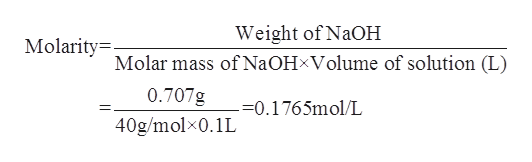# A chemist dissolves 707.mg of pure sodium hydroxide in enough water to make up 100.mL of solution. Calculate the pH of the solution. (The temperature of the solution is 25°C.) Be sure your answer has the correct number of significant digits.

Question
193 views

A chemist dissolves 707.mg of pure sodium hydroxide in enough water to make up 100.mL of solution. Calculate the pH of the solution. (The temperature of the solution is 25°C.) Be sure your answer has the correct number of significant digits.

check_circle

Step 1

The term pH of the solution is a measure of the acidity or the basicity of a given solution. More the pH of the solution, more will be its basicity. Lesser the value of pH of the solution, more will be the acidic strength of the solution. The formula to calculate the pH o a given solution is as follows:

Step 2

The weight of sodium hydroxide is given to be 707 mg=0.707 g.

The volume of the solution is given to be 100 mL = 0.1 L.

Molecular weight of NaOH =40g/mol

The molarity of the sodium hydroxide solution can be calculated as follows:help_outlineImage TranscriptioncloseWeight of NaOH Molarity= Molar mass of NaOHxVolume of solution (L) 0.707g =0.1765mol/L 40g/molx0.1L fullscreen
Step 3

1M of NaOH gives 1 M of hydroxide ions.

Therefore 0.1765 M ...

### Want to see the full answer?

See Solution

#### Want to see this answer and more?

Solutions are written by subject experts who are available 24/7. Questions are typically answered within 1 hour.*

See Solution
*Response times may vary by subject and question.
Tagged in

### Physical Chemistry